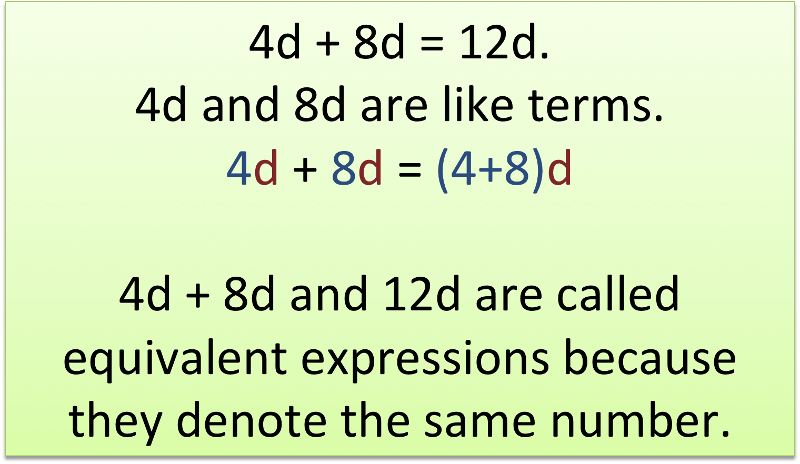# Like Terms

Recall that a term is a number, a variable, or a product or quotient of numbers and variables.   Like termsare terms that contain the same variable, with corresponding variables having the same power.

3x2+2x5has 3 distinct terms, no like terms2y2+6y2+5yhas 3 terms and 2 of them are like terms.   We can simplify this expression by combining the like terms, 2y2 and 6y2 to get 8y2+5y.

Example:

The distributive propertyand the properties of equality can be used to show thatAn expression is in simplest form when it is replaced by an equivalent expression having no like terms or parentheses.

## Practice

Evaluate each expression for the given value of the variable.
1. 8m – 5 for m = 9
2. 3n + 4 for n = 3
3. y ÷ 7 for y = 49
4. k x 4 – 6 for k = 3

Write each phrase as a numerical or algebraic expression.
5. 26 more than n
6. the product of 6 and 5

Simplify each expression.
7. 5 + 3x + 2y + x – 7 + 4y
8. 3x2 + 18 – 3x + 3x2 + 9x + 5x
9. -2x + x2 – x + 4x2 + 6x2 + 8x + 1
10. 2x + 4x + 7 + 4
11. 4x – 10 – x – 1
12. 9x + 9x – 2 – 6 – 9x
13. 7xy – 3xy + 4
14. 7x + 2y + 5x + 9y + 3x

(source)